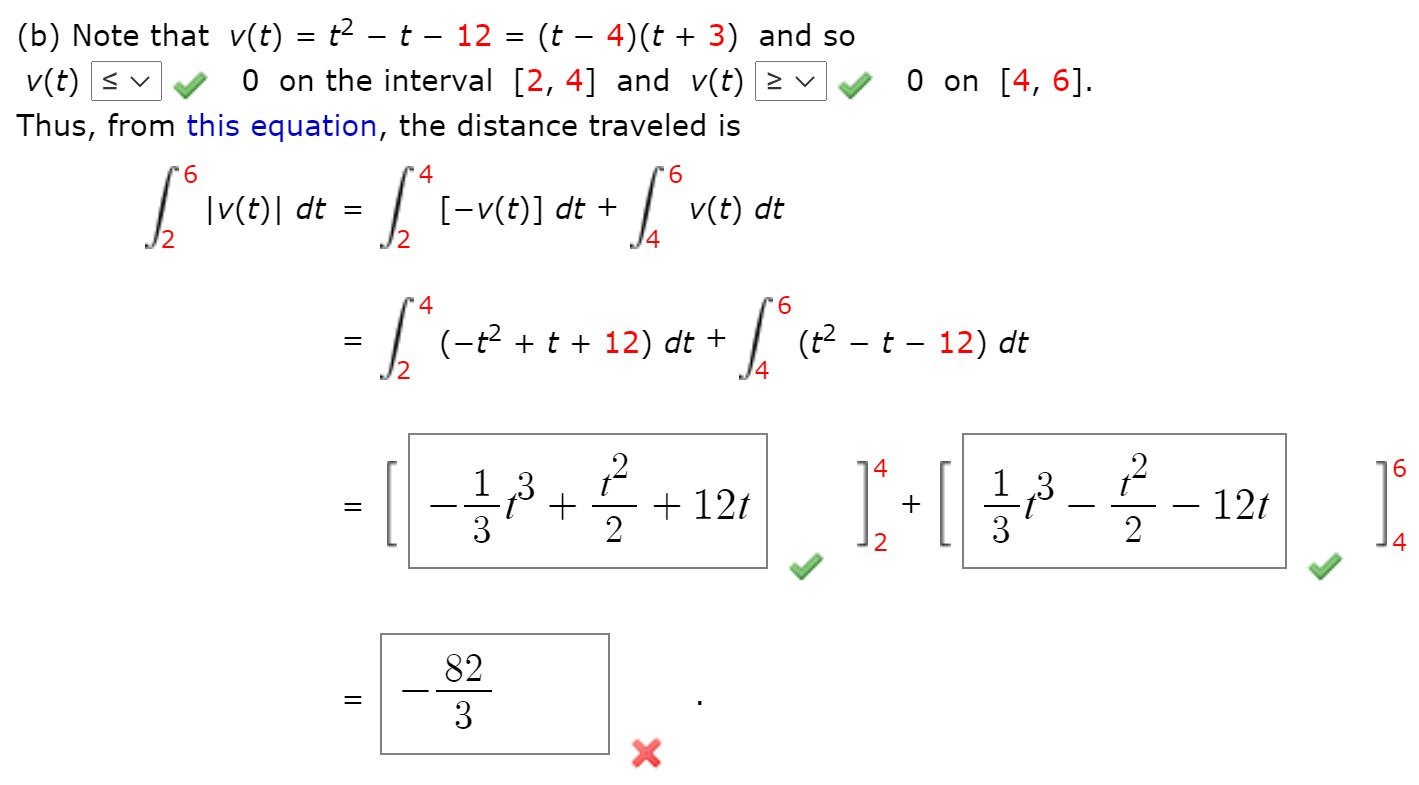# (b) Note that v(t) = t² – t – 12 = (t – 4)(t + 3) and so O on the interval [2, 4] and v(t) > v %3| v(t) < v O on [4, 6]. Thus, from this equation, the distance traveled is 4 9. |v(t)| dt [-v(t)] dt + v(t) dt 4. 4 9. = | (-?+t + 12) | (? - t - 12) dt dt + 16 ,3 + 12t - 12t 2 + 2 14 82 3

Question

A particle moves along a line so that its velocity at time t is v(t)=t2-t-12. Find the distance traveled during this time period. (Please see the image!) Thank you!!help_outlineImage Transcriptionclose(b) Note that v(t) = t² – t – 12 = (t – 4)(t + 3) and so O on the interval [2, 4] and v(t) > v %3| v(t) < v O on [4, 6]. Thus, from this equation, the distance traveled is 4 9. |v(t)| dt [-v(t)] dt + v(t) dt 4. 4 9. = | (-?+t + 12) | (? - t - 12) dt dt + 16 ,3 + 12t - 12t 2 + 2 14 82 3 fullscreen

## Expert Answer

### Want to see this answer and more?

Experts are waiting 24/7 to provide step-by-step solutions in as fast as 30 minutes!*

*Response times may vary by subject and question complexity. Median response time is 34 minutes for paid subscribers and may be longer for promotional offers.
Tagged in
Math
Calculus

### Integration© 2021 bartleby. All Rights Reserved.